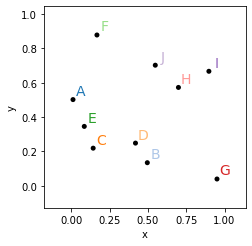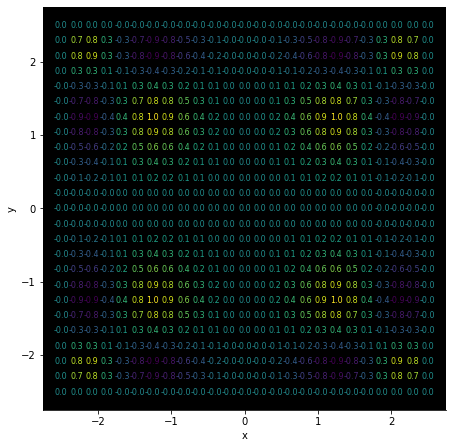# Labels#

Title
Labels Element
Dependencies
Matplotlib
Backends
Matplotlib
Bokeh
```import numpy as np
import holoviews as hv
from holoviews import opts

hv.extension('matplotlib')
```The `Labels` element may be used to annotate a plot with a number of labels. Unlike the `Text` element, `Labels` is vectorized and allows plotting many labels at once. It also supports any tabular or gridded data format. This also means that most other elements may be cast to a `Labels` element to annotate or label the values.

`Labels` also support various options that make it convenient to use as an annotation, e.g. `xoffset` and `yoffset` options allow adjusting the position of the labels relative to an existing data point and the `color` option allows us to colormap the data by a certain dimension.

```np.random.seed(9)
data = np.random.rand(10, 2)
points = hv.Points(data)
labels = hv.Labels({('x', 'y'): data, 'text': [chr(65+i) for i in range(10)]}, ['x', 'y'], 'text')

(points* labels).opts(
opts.Labels(color='text', cmap='Category20', xoffset=0.05, yoffset=0.05, size=14, padding=0.2),
opts.Points(color='black', s=25))
```If the value dimension of the data is not already of string type it will be formatted using the applicable entry in `Dimension.type_formatters` or an explicit `value_format` defined on the Dimension. Additionally the `color_index` option allows us to colormap the text by a dimension.

Here we will create a 2D array of values, define a Dimension with a formatter and then colormap the text:

```value_dimension = hv.Dimension('Values', value_format=lambda x: '%.1f' % x)
xs = ys = np.linspace(-2.5, 2.5, 25)
zs = np.sin(xs**2)*np.sin(ys**2)[:, np.newaxis]

hv.Labels((xs, ys, zs), vdims=value_dimension).opts(
opts.Labels(bgcolor='black', cmap='viridis', color='Values', fig_size=200, padding=0.05, size=8))
```This web page was generated from a Jupyter notebook and not all interactivity will work on this website. Right click to download and run locally for full Python-backed interactivity.